# Algebra for ALLPage 1

#### WATCH ALL SLIDES

Slide 1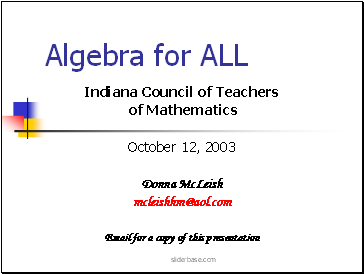Algebra for ALL

Indiana Council of Teachers

of Mathematics

October 12, 2003

Donna McLeish

mcleishhm@aol.com

Email for a copy of this presentation

sliderbase.com

Slide 2The FEAR of Algebra

New Content in Math Textbooks

sliderbase.com

Slide 3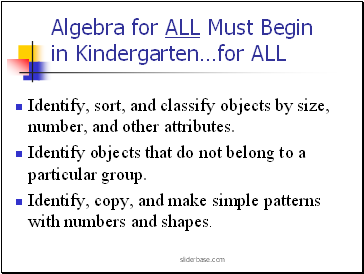## Algebra for ALL Must Begin in Kindergarten…for ALL

Identify, sort, and classify objects by size, number, and other attributes.

Identify objects that do not belong to a particular group.

Identify, copy, and make simple patterns with numbers and shapes.

sliderbase.com

Slide 4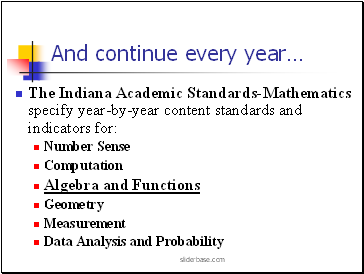## And continue every year…

The Indiana Academic Standards-Mathematics specify year-by-year content standards and indicators for:

Number Sense

Computation

Algebra and Functions

Geometry

Measurement

Data Analysis and Probability

sliderbase.com

Slide 5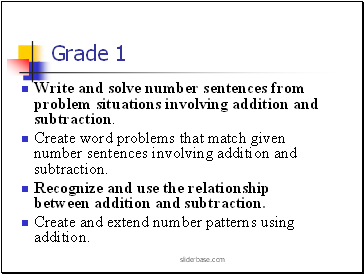Write and solve number sentences from problem situations involving addition and subtraction.

Create word problems that match given number sentences involving addition and subtraction.

Recognize and use the relationship between addition and subtraction.

Create and extend number patterns using addition.

sliderbase.com

Slide 6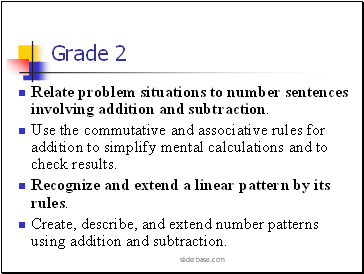Relate problem situations to number sentences involving addition and subtraction.

Use the commutative and associative rules for addition to simplify mental calculations and to check results.

Recognize and extend a linear pattern by its rules.

Create, describe, and extend number patterns using addition and subtraction.

sliderbase.com

Slide 7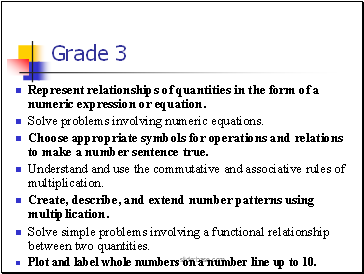Represent relationships of quantities in the form of a numeric expression or equation.

Solve problems involving numeric equations.

Choose appropriate symbols for operations and relations to make a number sentence true.

Understand and use the commutative and associative rules of multiplication.

Create, describe, and extend number patterns using multiplication.

Solve simple problems involving a functional relationship between two quantities.

Plot and label whole numbers on a number line up to 10.

sliderbase.com

Slide 8